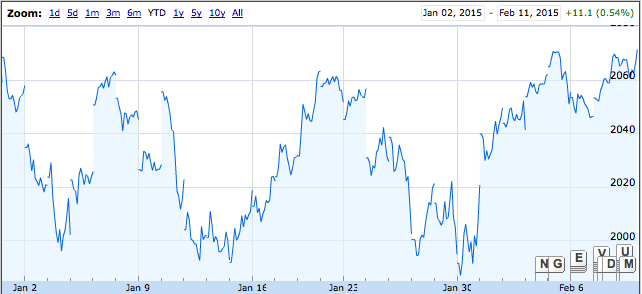# Historically speakingHow is historical trading-day volatility measured?

Step 1:
A) Calculate the absolute change in closing prices as the data set.
B) Calculate the percentage change in closing prices as the data set.

Step 2:
C) Calculate the standard deviation of the data set.
D) Calculate the variance of the data set.

Step 3:
E) Multiply it by $\sqrt{ 252}$.
F) Multiply it by $\sqrt{365}$.